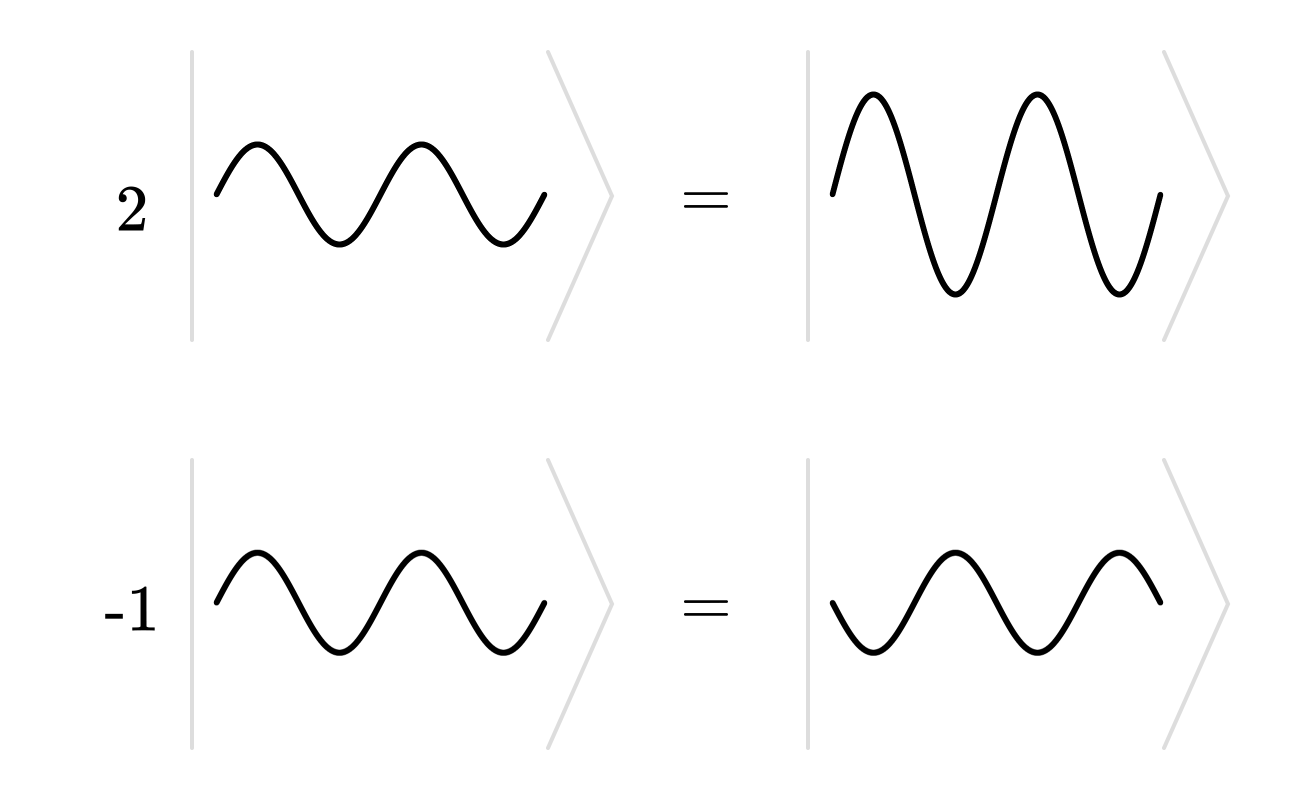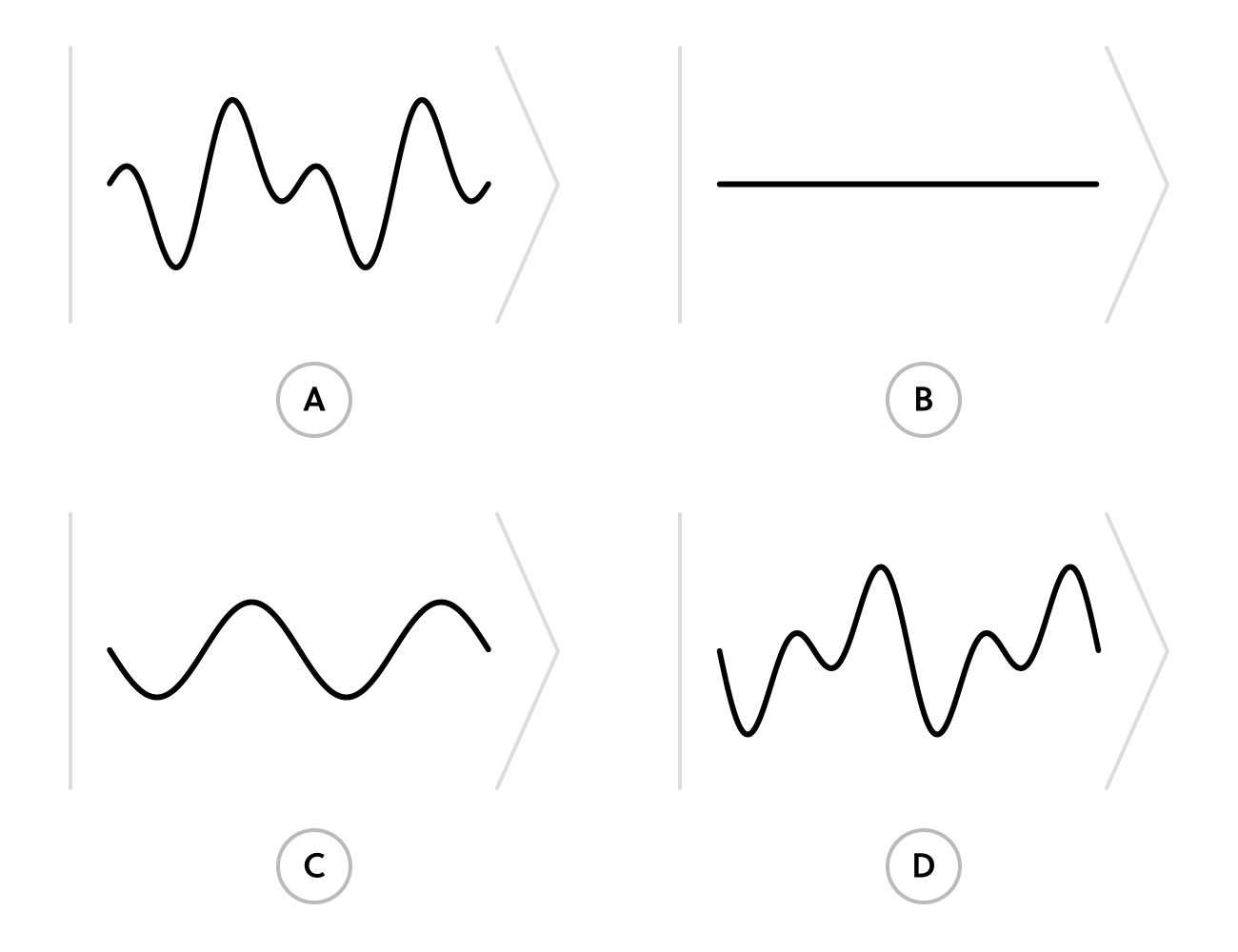### Linear Algebra with Applications

Waves are all around us. Light waves bounce off objects and into our eyes, allowing us to see; compression waves in the air allow us to hear.

It turns out that waves and the geometric arrows of the last quiz have a lot in common: they're both vectors!# Waves as Abstract Vectors

The notation from the last page is called a ket. It's a “wrapper” used to indicate that an object is a vector by writing $\text{“object”}$ as $\ket{\text{“object”}}.$

For example, the animation below "wraps" a few waves in ket notation:Waves wrapped in ket notation to emphasize their "vector" nature.

It drives home the idea that we're thinking of waves as vectors.

The equalities come from two general facts about function graphs:

• "doubling" a graph stretches it vertically by a factor of two, and
• multiplying by $-1$ flips the graph vertically.

We're free to put anything inside a ket so long as it comes from a set of objects that has all the properties of a vector space.

# Waves as Abstract Vectors

Let's be precise about how we define a vector space in ket notation:

A vector space $V$ is a non-empty set of objects $|v\rangle$ with some kind of addition and scalar multiplication that satisfy the following:

• Additive associativity: $|u\rangle + (|v\rangle + |w\rangle) = (|u\rangle + |v\rangle) + |w\rangle \\\\ \text{} \\\\$
• Multiplicative associativity: $a \big(b |v\rangle\big) = (ab) |v \rangle \\\\ \text{} \\\\$
• Additive commutativity: $|v\rangle + |w\rangle = |w\rangle + |v\rangle \\\\ \text{} \\\\$
• Existence of $|0\rangle$: $|v\rangle + |0\rangle = |v\rangle, \, |v\rangle + (-1) |v\rangle = |0 \rangle \\\\ \text{} \\\\$
• Multiplicative identity: $1 |v\rangle = |v\rangle$ for all elements in $V \\\\ \text{} \\\\$
• Scalar distributivity: $a\big(|v\rangle + |w\rangle\big) = a|v\rangle + a|w\rangle \\\\ \text{} \\\\$
• Vector distributivity: $(a+b)|v\rangle = a|v\rangle+ b|v\rangle$

(No need to memorize this list; we'll link back to it later when we need it.)

# Waves as Abstract Vectors

Let's prove that waves form a vector space by checking that all of the properties hold. First, let's define scalar multiplication and addition.

There's a natural way of scaling waves by (nonnegative) numbers:

Scaling a wave with amplitude $A$ by $c$ changes its amplitude to $c A.$

There's also a natural way of multiplying a wave by $-1:$

Scaling a wave by $-1$ flips it over vertically.

Describe the most sensible definition of scaling a wave by $c < 0.$Examples of the scaling rules for $c \geq 0$ and $c = -1,$ respectively

# Waves as Abstract Vectors

The very definition of scalar multiplication has multiplicative associativity and scalar distributivity built right in.

Now, let's show that our collection of waves has a zero vector.

Which option below makes the most sense for the $“0"$ wave?

Hint: We know that $0 \vec{v} = \vec{0},$ the arrow with no length, for any arrow $\vec{v}.$

What can we learn from this?

Options:# Waves as Abstract Vectors

Scalar multiplication is just one part of the story: we also need to add.

Mathematically, we add waves the same way we add the graphs of two functions. Physically, wave addition happens all the time.

When two tones combine, they make a beat; when light waves mix together in a film of oil, they interfere and make a rainbow pattern.

The interactive below adds some example wave pairs together. Press the play button to watch them oscillate:

# Waves as Abstract Vectors

Let's see if wave addition has the same properties as arrow addition.

Toggle through the various choices of waves; from what you can see, does wave addition seem commutative?

# Waves as Abstract Vectors

Since addition of functions is associative and commutative, so is wave addition. We only have a few more vector space properties to check!

Now let's mix scalar multiplication together with wave addition.

The interactive below demonstrates which two wave addition rules?

# Waves as Abstract Vectors

Select one or more

Experiment with different scalars $c$ below.

Which vector property (distributivity, associativity, commutativity) is being displayed in the interactive?

# Waves as Abstract Vectors

We've gone through the complete list of properties and verified that the set of waves is, in fact, a vector space.

So putting waves inside of kets throughout the quiz was totally justified!

You may wonder what good is done by recognizing waves as vectors.

If we can show that something is true as a consequence of the rules of vector spaces, then it will be true for any example of a vector.

This is the power of vector spaces.

It means that tools developed for use in, say, geometry carry over to far-flung applications like artificial intelligence and quantum mechanics.

The next quiz will give us a sense of how this works with a very important problem from signals analysis.

# Waves as Abstract Vectors

×